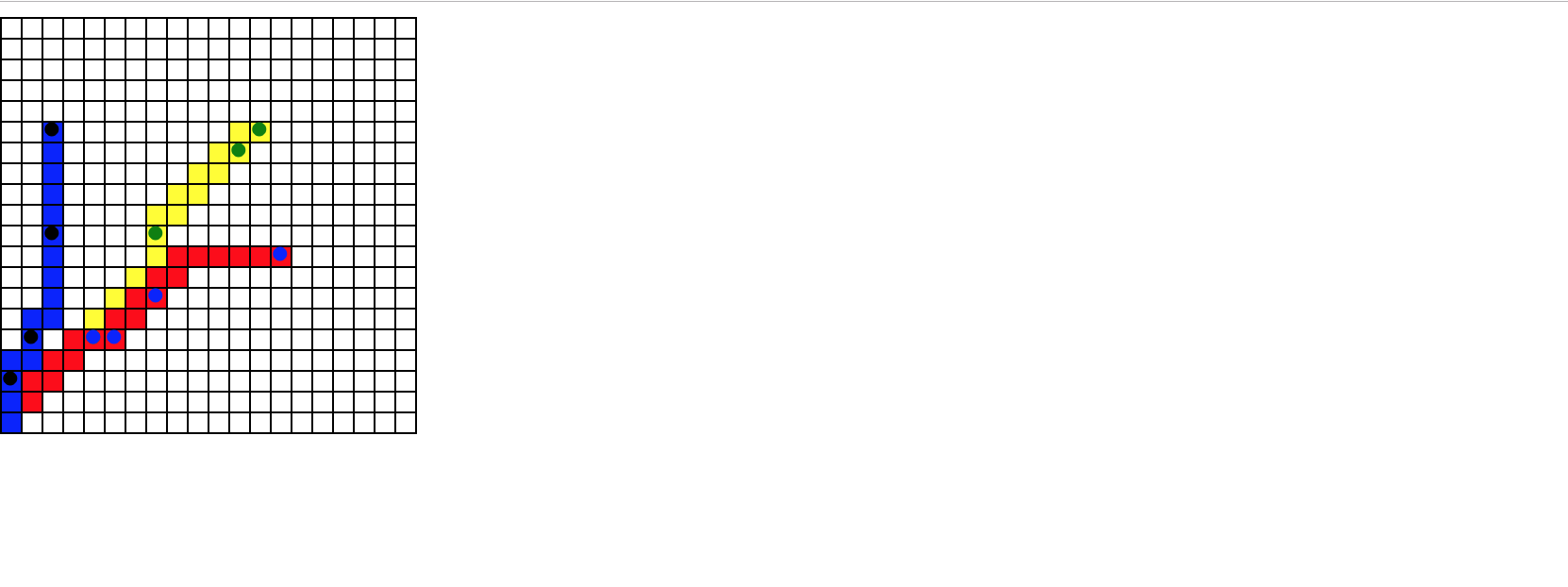# react-graph-n-plot

A graph component for inserting into react projects.

## Usage no npm install needed!

``````<script type="module">
import reactGraphNPlot from 'https://cdn.skypack.dev/react-graph-n-plot';
</script>``````

# react-graph-n-plot

A graph component for inserting into react projects. Useful for small to medium size graphs.

## Install

``````npm install --save react-graph-n-plot

import Graph from 'react-graph-n-plot'
``````

## Usage

Import the graph component and use it with props. Each object inside the `plotSets` object will create a separate line. To graph only a single set of points, have only a single object inside `plotSets`.

``````const plotSets = [
{
plots: [{ x: 5, y: 5 }, { x: 8, y: 10 }, { x: 12, y: 14 }, { x: 13, y: 15 }],
plotColor: 'green',
lineColor: 'yellow'
},
{
plots: [{ x: 5, y: 5 }, { x: 6, y: 5 }, { x: 8, y: 7 }, { x: 14, y: 9 }],
plotColor: 'blue',
lineColor: 'red'
},
{
plots: [{ x: 1, y: 3 }, { x: 2, y: 5 }, { x: 3, y: 10 }, { x: 3, y: 15 }],
plotColor: 'black',
lineColor: 'blue'
}
]
export default class App extends Component {
render() {
return <Graph setGraphSize={{ x: 20, y: 20 }} plotSets={plotSets} />
}
}
``````

### Props

The `Graph` takes two props.

1. setGraphSize - Object: sets the size of the graph. Both x and y must be equal.

2. plotSets - Object: contains one or many objects with the data used for plotting the points and connecting them to each other.

• plots - Array: an array of objects. Each object is the x/y coords of a plot point.
• plotColor - String: a string designating the color of the plot points. Leave blank for no visible plot points.
• lineColor - String: a string designating the color of the line being graphed. Leave blank for no line color.